# Gaussian process introductory tutorial in Python¶

by: Andreas Damianou - andreasdamianou.com

Latest update: October 2019

You can also see this notebook rendered in nbviewer [LINK].

If you wish to cite this tutorial:

% Requires \usepackage{url} in preamble
@misc{DamianouGPtutorial,
author = {Damianou, Andreas},
title = {Gaussian process introductory tutorial in Python},
originalyear = {2019},
lastchecked = {}
}

Import necessary libraries and modules

In :
%pylab inline

import matplotlib.pyplot as plt
import matplotlib.mlab as mlab
import numpy as np
import scipy as sp
from scipy.stats import multivariate_normal

Populating the interactive namespace from numpy and matplotlib


The following function will be handy for plotting the fit of a GP.

In :
def plot_fit(x,y,mu,var, m_y='k-o', m_mu='b-<', l_y='true', l_mu='predicted', legend=True, title=''):
"""
Plot the fit of a GP
"""
if y is not None:
plt.plot(x,y, m_y, label=l_y)
plt.plot(x,mu, m_mu, label=l_mu)
vv = 2*np.sqrt(var)
plt.fill_between(x[:,0], (mu-vv)[:,0], (mu+vv)[:,0], alpha=0.2, edgecolor='gray', facecolor='cyan')
if legend:
plt.legend()
if title != '':
plt.title(title)


# Part 1: GP teaser¶

Let's have a quick look at some of the things one can do with GPs.

In :
import GPy
import pods # For the datasets
from sklearn.preprocessing import PolynomialFeatures
from sklearn.linear_model import LinearRegression
from sklearn import linear_model

#--- Data
y_train = pods.datasets.olympic_100m_men()['Y']
# Standardize data
y_train -= y_train.mean()
y_train /= y_train.std()
x_train = np.linspace(-1, 1, y_train.shape)[:,None]
x_test_left  = np.linspace(-2.5, -1, 9)[:,None] # Extrapolation data from the left
x_test_right = np.linspace(1, 2.5, 9)[:,None]   # Extrapolation data to the right

#--- Fit a polynomial model and predict inter/extrapolations
poly = PolynomialFeatures(degree = 3)
x_poly_train = poly.fit_transform(x_train)
lin_poly = LinearRegression()
lin_poly.fit(x_poly_train, y_train)

#--- Fit a GP model and predict inter/extrapolations
m = GPy.models.GPRegression(x_train, y_train)
K = m.kern.K(x_train, x_train).copy()
m.optimize()
y_gp, y_gp_std     = m.predict(x_train)
y_gp_l, y_gp_std_l = m.predict(x_test_left)
y_gp_r, y_gp_std_r = m.predict(x_test_right)

In :
#---- Interpolation with Polynomial model
plt.figure(figsize=(15,4))
plt.suptitle('Polynomial model', fontsize=12)
plt.subplot(1,2,1)
plt.scatter(x_train, y_train, color = 'blue', label="true data")
plt.plot(x_train, lin_poly.predict(x_poly_train), color = 'red', label="interpolation (poly)")
plt.legend()

#--- Extrapolation with Polynomial model
plt.subplot(1,2,2)
plt.scatter(x_train, y_train, color = 'blue', label="true data")
plt.plot(x_train, lin_poly.predict(x_poly_train), color = 'red', label="interpolation (poly)")
plt.plot(x_test_left, lin_poly.predict(poly.fit_transform(x_test_left) ), color='green', label="extrapolation (poly)")
plt.plot(x_test_right, lin_poly.predict(poly.fit_transform(x_test_right) ), color='green')
plt.legend()

plt.figure(figsize=(15,8))
plt.suptitle('GP model', fontsize=12)

#--- Interpolation with GP model
plt.subplot(2,2,1)
plot_fit(x_train, y_train, y_gp, y_gp_std, 'bo', 'r-', 'true data', 'interpolation(GP)')

#--- Extrapolation with GP model
plt.subplot(2,2,2)
plot_fit(x_train, y_train, y_gp, y_gp_std, 'bo', 'r-', 'true data', 'interpolation (GP)')
plot_fit(x_test_left, None, y_gp_l, y_gp_std_l, 'bo', 'g-', '', 'extrapolation (GP)')
plot_fit(x_test_right, None, y_gp_r, y_gp_std_r, 'bo', 'g-', legend=False)

#---- Plot *prior* samples from GP model
plt.subplot(2,2,3)
x_samp = np.linspace(x_test_left, x_test_right[-1], 20)[:,None]
N_samp = 5
prior_samp = np.zeros((N_samp, x_train.shape))
for i in np.arange(N_samp):
prior_samp[i,:] = np.random.multivariate_normal(mean=np.zeros(x_train.shape), cov=K)
plt.plot(prior_samp[i,:], label='prior_samp_'+str(i))
plt.legend()

#--- Plot *posterior* samples from GP model
plt.subplot(2,2,4)
plot_fit(x_samp, None,  m.predict(x_samp), m.predict(x_samp), 'bo', 'g-','','', legend=False)
plt.scatter(x_train, y_train, color = 'blue')
f_samp = m.posterior_samples_f(x_samp, 5)
for i in range(f_samp.shape):
plt.plot(x_samp, f_samp[:,:,i], label='post_sample_' + str(i))
plt.legend()

Out:
<matplotlib.legend.Legend at 0x1a1e2a2eb8>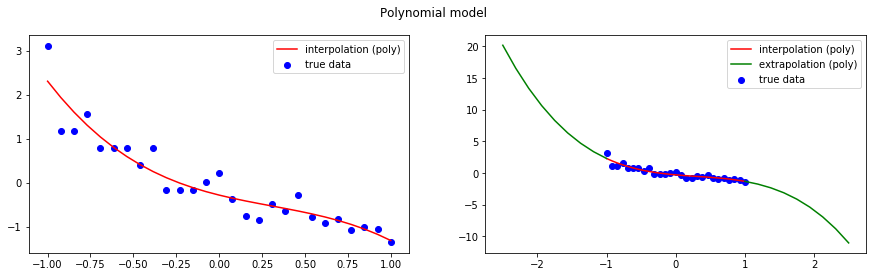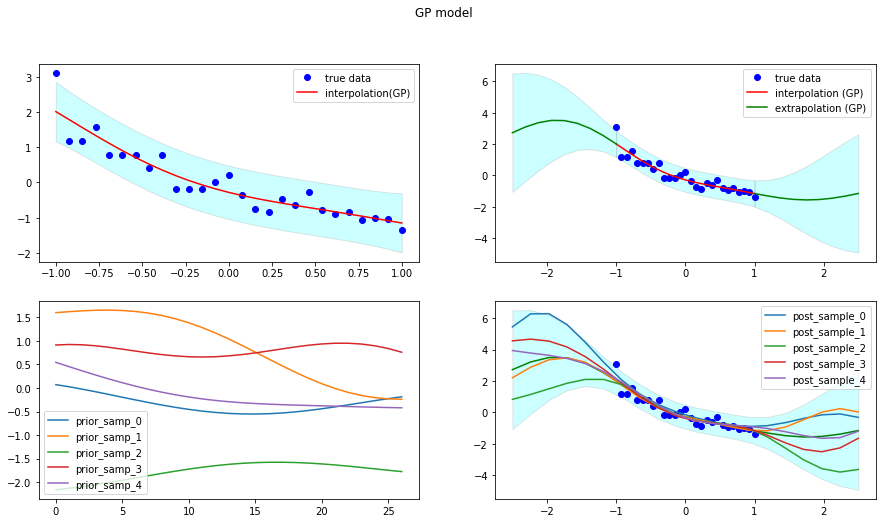#### Observations:¶

• The GP model gives uncertainty in the fit/predictions, shown as shaded area.
• The GP model is, naturally, more uncertain in extrapolations.
• The GP model, away from the training data, reverts to its prior (here implicitly zero, that can be changed) rather than confidently giving unrealistic predictions.
• The GP model can be investigated before training (prior samples) or after training (posterior samples). The samples tell us "what sort of functions does that model consider".

# Part 2: GPs as infinite dimensional Gaussian distributions¶

A Gaussian process (GP) is a collection of random variables, any finite number of which have a joint Gaussian distribution.

Equivalently, a GP can be seen as a stochastic process which corresponds to an infinite dimensional Gaussian distribution.

## Intuition by sampling and plotting Gaussians¶

Let's first define some plotting functions that we'll use later.

In :
def gen_Gaussian_samples(mu, sigma, N=200):
"""
Generate N samples from a multivariate Gaussian with mean mu and covariance sigma
"""
D = mu.shape
samples = np.zeros((N,D))
for i in np.arange(N):
samples[i,:] = np.random.multivariate_normal(mean=mu, cov=sigma)
return samples.copy()

def gen_plot_Gaussian_samples(mu, sigma,N=1000):
"""
Generate N samples from a multivariate Gaussian with mean mu and covariance sigma
and plot the samples as they're generated
"""
for i in np.arange(N):
sample = np.random.multivariate_normal(mean=mu, cov=sigma)
plt.plot(sample,sample, '.',color='r',alpha=0.6)
plt.grid()

def plot_Gaussian_contours(x,y,mu,sigma,N=100):
"""
Plot contours of a 2D multivariate Gaussian based on N points. Given points x and y are
given for the limits of the contours
"""
X, Y = np.meshgrid(np.linspace(x.min()-0.3,x.max()+0.3,100), np.linspace(y.min()-0.3,y.max()+0.3,N))
rv = multivariate_normal(mu, sigma)
Z = rv.pdf(np.dstack((X,Y)))
plt.contour(X,Y,Z)
plt.xlabel('x_1')
plt.ylabel('x_2')

def plot_sample_dimensions(samples, colors=None, markers=None, ms=10):
"""
Given a set of samples from a bivariate Gaussian, plot them, but instead of plotting them
x1 vs x2, plot them as [x1 x2] vs ['1', '2']
"""
N = samples.shape
D = samples.shape

t=np.array(range(1,D+1))

for i in np.arange(N):
if colors is None and markers is None:
plt.plot(t,samples[i,:], '-o',ms=ms)
elif colors is None:
plt.plot(t,samples[i,:], '-o',marker=markers[i],ms=ms)
elif markers is None:
plt.plot(t,samples[i,:], '-o',color=colors[i],ms=ms)
else:
plt.plot(t,samples[i,:], '-o',color=colors[i],marker=markers[i],ms=ms)
plt.grid()
plt.xlim([0.8,t[-1]+0.2])
plt.ylim([samples.min()-0.3, samples.max()+0.3])
plt.xlabel('d = {' + str(t) + '}')
plt.ylabel('[x_d]')
plt.gca().set_title(str(N) + ' samples from a bivariate Gaussian')

def set_limits(samples):
plt.xlim([samples[:,0].min()-0.3, samples[:,0].max()+0.3])
plt.ylim([samples[:,1].min()-0.3, samples[:,1].max()+0.3])


Test the two different ways of plotting a bivariate Gaussian.

In :
colors = ['r','g','b','m','k']
markers = ['p','d','o','v','<']

N=5 # Number of samples
mu = np.array([0,0])  # Mean of the 2D Gaussian
sigma = np.array([[1, 0.5], [0.5, 1]]); # covariance of the Gaussian

# Generate samples
samples = gen_Gaussian_samples(mu,sigma,N)

f=figure(figsize=(12,12));
ax1=plt.subplot(1, 2, 1,autoscale_on=False, aspect='equal')
set_limits(samples)
plot_Gaussian_contours(samples[:,0],samples[:,1],mu,sigma)

# Plot samples
for i in np.arange(N):
plt.plot(samples[i,0],samples[i,1], 'o', color=colors[i], marker=markers[i],ms=10)
plt.grid()
plt.gca().set_title(str(N) + ' samples of a bivariate Gaussian.')

ax2=plt.subplot(1, 2, 2,autoscale_on=False, aspect='equal')
plot_sample_dimensions(samples=samples, colors=colors, markers=markers)
#ax2.set(autoscale_on=False, aspect='equal')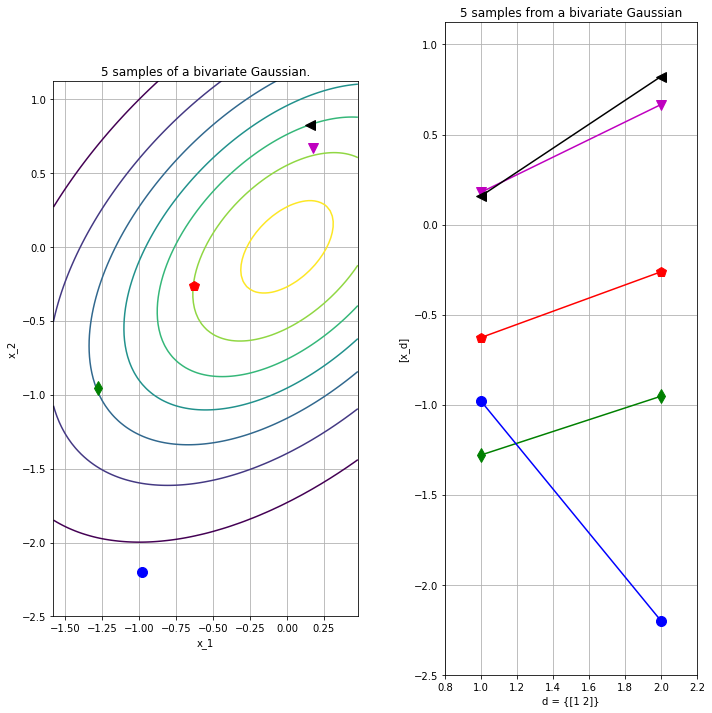Repeat as before, but now we'll plot many samples from two kinds of Gaussians: one which with strongly correlated dimensions and one with weak correlations

In :
# Plot with contours. Compare a correlated vs almost uncorrelated Gaussian

sigmaUncor = np.array([[1, 0.02], [0.02, 1]]);
sigmaCor = np.array([[1, 0.95], [0.95, 1]]);

f=figure(figsize=(15,15));

ax=plt.subplot(1, 2, 1); ax.set_aspect('equal')
samplesUncor=gen_Gaussian_samples(mu,sigmaUncor)
plot_Gaussian_contours(samplesUncor[:,0],samplesUncor[:,1], mu, sigmaUncor)
gen_plot_Gaussian_samples(mu, sigmaUncor)
plt.gca().set_title('Weakly correlated Gaussian')

ax=plt.subplot(1, 2, 2); ax.set_aspect('equal')
samplesCor=gen_Gaussian_samples(mu,sigmaCor)
plot_Gaussian_contours(samplesCor[:,0],samplesCor[:,1], mu, sigmaCor)
gen_plot_Gaussian_samples(mu, sigmaCor)
plt.gca().set_title('Stongly correlated Gaussian')

Out:
Text(0.5,1,'Stongly correlated Gaussian')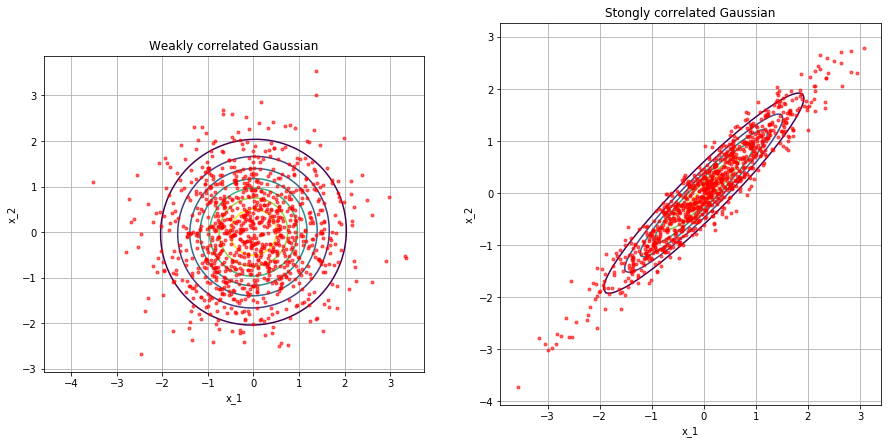In :
# But let's plot them as before dimension-wise...

f=figure(figsize=(18,5));
perm = np.random.permutation(samplesUncor.shape)[0::14]

ax1=plt.subplot(1, 2, 1); ax1.set_aspect('auto')
plot_sample_dimensions(samplesUncor[perm,:])
plt.gca().set_title('Weakly correlated')
ax2=plt.subplot(1, 2, 2,sharey=ax1); ax2.set_aspect('auto')
plot_sample_dimensions(samplesCor[perm,:])
plt.gca().set_title('Strongly correlated')
plt.ylim([samplesUncor.min()-0.3, samplesUncor.max()+0.3])

Out:
(-4.5995273486559585, 3.1053517856856994)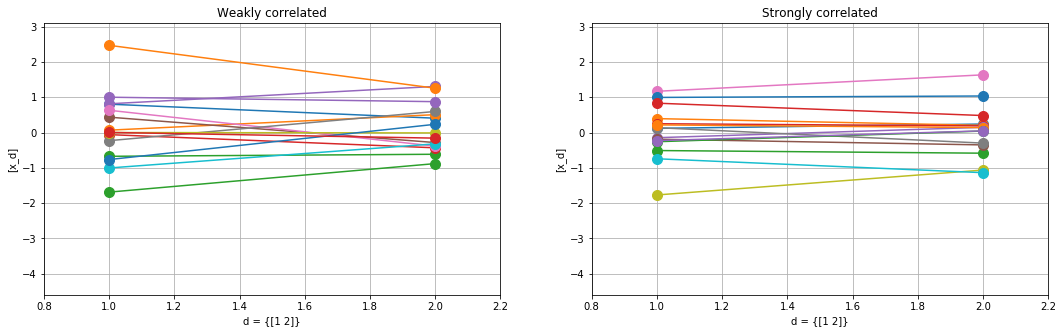The strongly correlated Gaussian results in more "horizontal" lines in the dimension-wise plot.

More importantly, by using the dimension-wise plot, we are able to plot Gaussians which have more than two dimensions. Below we plot N samples from a D=8-dimensional Gaussian. Because I don't want to write down the full 8x8 covariance matrix, I define a "random" one through a mathematical procedure that is guaranteed to give me back a positive definite and symmetric matrix (i.e. a valid covariance). More on this later.

In :
N=5
mu = np.array([0,0,0,0,0,0,0,0])
D = mu.shape

# Generate random covariance matrix
tmp = np.sort(sp.random.rand(D))[:,None]
tmp2 = tmp**np.arange(5)
sigma = 5*np.dot(tmp2,tmp2.T) + 0.005*np.eye(D)

samples = gen_Gaussian_samples(mu,sigma,N)

for i in np.arange(N):
plt.plot(tmp,samples[i,:], '-o')
plt.grid()

plt.gca().set_title(str(N) + ' samples of a ' + str(D) + ' dimensional Gaussian')

Out:
Text(0.5,1,'5 samples of a 8 dimensional Gaussian')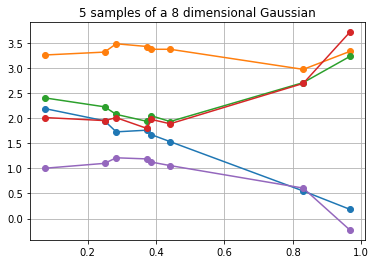Taking this even further, we can plot samples from a 200-dimensional Gaussian in the dimension-wise plot.

In :
N=5
D=200
mu = np.zeros((D,1))[:,0]

# Generate random covariance matrix
tmp = np.sort(sp.random.rand(D))[:,None]
tmp2 = tmp**np.arange(5)
sigma = 5*np.dot(tmp2,tmp2.T)+ 0.0005*np.eye(D)

samples = gen_Gaussian_samples(mu,sigma,N)

for i in np.arange(N):
plt.plot(tmp,samples[i,:], '-')

plt.gca().set_title(str(N) + ' samples of a ' + str(D) + ' dimensional Gaussian')

Out:
Text(0.5,1,'5 samples of a 200 dimensional Gaussian')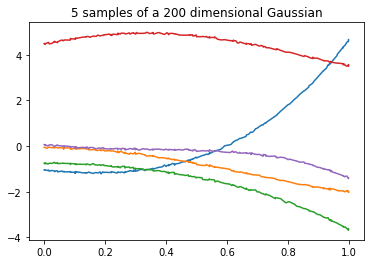We see that each sample now starts looking like a "smooth" curve. Therefore, we now have a clear intuition as to why a GP can be seen as an infinite dimensional multivariate Gaussian which is used as a prior over functions, since one sample from a GP is a function.

### Mean and covariance function¶

Similarly to how a D-dimensional Gaussian is parameterized by its mean vector and its covariance matrix, a GP is parameterized by a mean function and a covariance function. To explain this, we'll assume (without loss of generality) that the mean function is $\mu(x) = \mathbf{0}$. As for the covariance function, $k(x,x')$, it is a function that receives as input two locations $x,x'$ belonging to the input domain, i.e. $x,x' \in \mathcal{X}$, and returns the value of their co-variance.

In this way, if we have a finite set of input locations we can evaluate the covariance function at every pair of locations and obtain a covariance matrix $\mathbf{K}$. We write: $$\mathbf{K} = k(\mathbf{X}, \mathbf{X}),$$ where $\mathbf{X}$ is the collection of training inputs.

More on covariance functions later. For the moment, think of them as kind of a black box.

Importantly, even if we assume that the input domain is inifinte, e.g. $\mathbb{R}$, we can get away with never having to perform infinite number of operations. This is because of the marginalization property of the Gaussian distribution. See below.

## Marginalization and conditioning properties of the Gaussian¶

### Joint¶

Let's start with a multivariate Gaussian. Assume that we have a random variable $\mathbf{f}$ which follows a multivariate Gaussian, and we partition its dimensions into two sets, $A,B$. Then, the joint distribution can be written as: $$p(\underbrace{f_1, f_2, \cdots, f_s}_{\mathbf{f}_A}, \underbrace{f_{s+1}, f_{s+2}, \cdots, f_N}_{\mathbf{f}_B}) \sim \mathcal{N}(\boldsymbol \mu, \mathbf{K}).$$ with: $$\boldsymbol \mu = \begin{bmatrix} \boldsymbol \mu_A \\ \boldsymbol \mu_B \end{bmatrix} \; \; \text{and} \; \; \mathbf{K} = \begin{bmatrix} \mathbf{K}_{A A} & \mathbf{K}_{A B} \\ \mathbf{K}_{B A} & \mathbf{K}_{B B} \end{bmatrix}$$

### Marginal¶

And the marginal distribution can be written as:

$$p(\mathbf{f}_A, \mathbf{f}_B) \sim \mathcal{N}(\boldsymbol \mu, \mathbf{K}). \text{ Then:} \\ p(\mathbf{f}_A) = \int_{\mathbf{f}_B} p(\mathbf{f}_A, \mathbf{f}_B) \text{d} \mathbf{f}_B = \mathcal{N}(\boldsymbol \mu_A, \mathbf{K}_{A A}) %\\ % p(\mathbf{f}_B) = \int_{\mathbf{f}_A} p(\mathbf{f}_A, \mathbf{f}_B) \text{d} \mathbf{f}_A = % \mathcal{N}(\boldsymbol \mu_B,\mathbf{K}_{B B})$$

The marginalization property means that the training data that have and any (potentially infinite in number) test data $f_*$ that we have not seen (yet), follow a (potentially infinite) Gaussian distribution with mean and covariance:

$$\boldsymbol \mu_{\infty} = \begin{bmatrix} \boldsymbol \mu_{\!_\mathbf{X}} \\ \cdots \\ \cdots \end{bmatrix} \; \; \text{and} \; \; \mathbf{K}_{\infty} = \begin{bmatrix} \mathbf{K}_{\!_\mathbf{X} \!_\mathbf{X}} & \cdots \\ \cdots & \cdots \end{bmatrix}$$

where $\mathbf{X}$ is training inputs and $\mathbf{K}_{XX}$ is the covariance matrix constructing by evaluating the covariance function at all given inputs.

So, in the Gaussian process case (assuming 0 mean) we have a joint Gaussian distribution of the training and the (potentially infinite!) test data:

$$\begin{bmatrix}\mathbf{f} \\ \mathbf{f}^*\end{bmatrix} \sim \mathcal{N}\left(\mathbf{0}, \begin{bmatrix} \mathbf{K} & \mathbf{K}_\ast \\ \mathbf{K}_\ast^\top & \mathbf{K}_{\ast,\ast}\end{bmatrix}\right)$$

Here, $\mathbf{K}_\ast$ is the (cross)-covariance matrix obtained by evaluating the covariance function in pairs of training inputs $\mathbf{X}$ and test inputs $\mathbf{X_*}$, ie.

$$\mathbf{K}_\ast = k(\mathbf{X}, \mathbf{X}_*) .$$

Similarly:

$$\mathbf{K}_{\ast\ast} = k(\mathbf{X}_*, \mathbf{X}_*) .$$

### Conditional¶

Interestingly, conditioning a multivariate Gaussian to obtain the posterior distribution also yields a Gaussian: Again, if $$p(\mathbf{f}_A, \mathbf{f}_B) \sim \mathcal{N}(\boldsymbol \mu, \mathbf{K}). \; \; \text{Then:} \\ p(\mathbf{f}_A | \mathbf{f}_B) = \mathcal{N}(\boldsymbol \mu_A + \mathbf{K}_{AB} \mathbf{K}^{-1}_{BB} (\mathbf{f}_B - \boldsymbol \mu_B), \mathbf{K}_{AA} - \mathbf{K}_{AB}\mathbf{K}_{BB}^{-1}\mathbf{K}_{BA})% \\ % p(\mathbf{f}_B | \mathbf{f}_A) = \mathcal{N}(\cdots, \cdots)$$

In the GP context this can be used for inter/extrapolation. Assume that we have a function $f$ with input domain $\mathcal{X} = \mathbb{R}$ and we set a GP prior on $f$ (so, now we use $f$ to denote function evaluations, rather than random variables). Also assume that we have a training set $\mathbf{X} = [x_1, x_2, \dots x_N]$. Then, we can condition on the function ouputs evaluated on the training set in order to perform inference for the function value at any input location $x_* \in \mathbb{R}$. This conditioning means finding the GP posterior process:

$$p(\mathbf{f_*} | \mathbf{f_1}, \cdots, \mathbf{f_N}) = p(f(x_*) | f(x_1), \cdots, f(x_N)) \\ \sim \mathcal{N}(\mathbf{K}_*^\top \mathbf{K}^{-1} \mathbf{f}\; , \; \mathbf{K}_{*,*} - \mathbf{K}_*^\top \mathbf{K}^{-1} \mathbf{K}_*)$$

Remember, the test inputs $\mathbf{X}_*$ appear in the above expression inside $\mathbf{K}_*$ and $\mathbf{K}_{**}$.

## Noise model¶

As is standard in probabilistic regression, we assume a noise model. We take:

$$y = f(x) + \epsilon$$

where:

$$f \sim \mathcal{GP}(0, k(x,x'))$$

and

$$\epsilon \sim \mathcal{N}(0,\sigma^2 I) \; \; \; \; \; \; \; \; \; (1)$$

where non-bold symbols now denote single elements from the training vectors.

The covariance function $k(x,x')$ is a function which takes as inputs pairs in the input domain and returns their co-variance. By denoting $k(\mathbf{X},\mathbf{X})$ we mean that we evaluate the covariance function in the whole training set, $\mathbf{X}$, and this gives us back a covariance matrix.

The assumption about Gaussian noise says that the training data $(x,y) \in (\mathbf{X}, \mathbf{Y})$ are related by a function $f$ whose output is then corrupted by Gaussian noise (i.e. we have noisy observations). The above construction, gives us the following probabilities: \begin{equation} p(\mathbf{y}|\mathbf{f}) = \mathcal{N}(\mathbf{y}|\mathbf{f}, \sigma^2 \mathbf{I}) \end{equation}

$$p(\mathbf{f}|\mathbf{x}) = \mathcal{N}(\mathbf{f}|\mathbf{0}, K_{ff}) = (2 \pi)^{n/2} |K_{ff}|^{-1/2} \exp\left( -\frac{1}{2} \mathbf{f}^T K_{ff} \mathbf{f} \right) \text{where:} K_{ff} = k(\mathbf{x},\mathbf{x}) \; \; \; \; (2)$$$$p(\mathbf{y}|\mathbf{x}) = \int p(\mathbf{y}|\mathbf{f})p(\mathbf{f}|\mathbf{x}) d\mathbf{f} = \mathcal{N}(\mathbf{y}|\mathbf{0},K_{ff}+\sigma^{2} \mathbf{I}) \; \; \; \; (3)$$

where the last quantity is called the marginal likelihood and is tractable because of our choice for noise $\epsilon$ which is normally distributed.

## Predictions¶

Now, for a test point $x_*$ we want to compute its output on the observed space, i.e. we want to compute $y_*$. Building on the noise model and the previously shown expressions, the posterior for the test outputs is given by:

$$\mathbf{y}^* | \mathbf{y}, \mathbf{x}, \mathbf{x_*} \sim \mathcal{N}(\boldsymbol \mu_{\text{pred}},\mathbf{K}_{\text{pred}}) \; \; \; \; (4)$$

with
$$\boldsymbol \mu_{\text{pred}} = \mathbf{K}_*^\top \left[\mathbf{K} + \sigma^2 \mathbf{I}\right]^{-1} \mathbf{y}$$ and $$\mathbf{K}_{\text{pred}} = \mathbf{K}_{*,*} - \mathbf{K}_*^\top \left[\mathbf{K} + \sigma^2 \mathbf{I}\right]^{-1} \mathbf{K}_*.$$

# Covariance functions, aka kernels¶

We saw above their role for creating covariance matrices from training inputs, thereby allowing us to work with finite when the domain is potentially infinite.

We'll see below that the covariance function is what encodes our assumption about the GP. By selecting a covariance function, we are making implicit assumptions about the shape of the function we wish to encode with the GP, for example how smooth it is.

Even if the covariance function has a parametric form, combined with the GP it gives us a nonparametric model. In other words, the covariance function is specifying the general properties of the GP function we wish to encode, and not a specific parametric form for it.

Below we define two very common covariance functions: The RBF (also known as Exponentiated Quadratic or Gaussian kernel) which is differentiable infinitely many times (hence, very smooth), and the linear one: $$k_{RBF}(\mathbf{x}_{i,:},\mathbf{x}_{j,:}) = \sigma^2 \exp \left( -\frac{1}{2\ell^2} \sum_{q=1}^Q (x_{i,q} - x_{j,q})^2\right)$$ where $Q$ denotes the dimensionality of the input space. Its parameters are: the lengthscale, $\ell$ and the variance $\sigma^2$.

$$k_{lin}(\mathbf{x}_{i,:},\mathbf{x}_{j,:}) = \sigma^2 \mathbf{x}_{i,:}^T \mathbf{x}_{j,:}$$

Its parameters is the variance $\sigma^2$.

Below, we will implement and investigate them.

## Defining covariance function forms¶

In :
def cov_linear(x,x2=None,theta=1):
if x2 is None:
return np.dot(x, x.T)*theta
else:
return np.dot(x, x2.T)*theta

def cov_RBF(x, x2=None, theta=np.array([1,1])):
"""
Compute the Euclidean distance between each row of X and X2, or between
each pair of rows of X if X2 is None and feed it to the kernel.
"""
variance = theta
lengthscale = theta
if x2 is None:
xsq = np.sum(np.square(x),1)
r2 = -2.*np.dot(x,x.T) + (xsq[:,None] + xsq[None,:])
r = np.sqrt(r2)/lengthscale
else:
x1sq = np.sum(np.square(x),1)
x2sq = np.sum(np.square(x2),1)
r2 = -2.*np.dot(x, x2.T) + x1sq[:,None] + x2sq[None,:]
r = np.sqrt(r2)/lengthscale

return variance * np.exp(-0.5 * r**2)


## Experimenting with covariance function parameters¶

In :
X = np.sort(np.random.rand(400, 1) * 6 , axis=0)

params_linear = [0.01, 0.05, 1, 2, 4, 10]
params_rbf    = [0.005, 0.1, 1, 2, 5, 12]
K = len(params_linear)

plt.figure(figsize=(25,25))
j=1
for i in range(K):
plt.subplot(K,2,j)
K_rbf = cov_RBF(X,X,theta=np.array([1,params_rbf[i]]))
plt.imshow(K_rbf)
plt.colorbar()
plt.gca().set_title('RBF (l=' + str(params_rbf[i]) + ')')

plt.subplot(K,2,j+1)
K_lin = cov_linear(X,X,theta=params_linear[i])
plt.imshow(K_lin)
plt.colorbar()
plt.gca().set_title('Lin (var=' + str(params_linear[i]) + ')')

j+=2

plt.suptitle('RBF (left) and Linear (right) cov. matrices created with different parameters', fontsize=20)

Out:
Text(0.5,0.98,'RBF (left) and Linear (right) cov. matrices created with different parameters')